# Length + direct relationship - math problems

#### Number of problems found: 77

• Oil crisis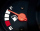A car runs 16 km using 1 litre of petrol. How much distance will it cover using 2 3/4 litres of petrol?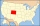The state Colorado has the shape of a rectangle. The map is sold on 2 posters. The first has dimensions of 70 cm and 50 cm in a scale of 1: 1,000,000. The second poster has dimensions of 175 cm and 125 cm. What is the scale of the second poster?
• The bricklayer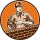The bricklayer built a six-meter base of the fence in 3 hours. How long does it take to build a 14 m long base?
• Clothes 4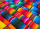If the cost of 16.5 metres of cloth is ₹2,062.50 then find the cost of 24.75 metres of clothes
• Two citiesOn a map, 1.5 cm represents 50 miles. If the distance between the two cities on the map is 6 cm, how many miles separate the cities?
• Petrol as fuelA car can travel 480 miles on a full tank of petrol. The tank holds 60 litres. The fuel gauge shows there are 15 litres left in the tank. How many more miles can the car travel before it runs out of petrol?
• A map 4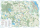A map represents every 4 miles with 1 inch. If a school and a bank are actually 12 miles apart, how far apart are they on the map, in inches?
• Mr Duma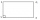Mr Duma recently inherited a rectangular plot, part of the estate left by his late father. The plot with the following dimensions: Length=2x+1;Width=x-1. Determine the formula, in terms of x, that best describes the area of the rectangular plot. He has pl
• Jewelry box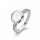The bottom of Zeyda's jewelry box is a rectangle with the length of 5 3/8 inches and a width of 3 1/4 inches. What is the area of the bottom of the jewelry box?
• Scale factor 2Nadeem has a 10 inch by 10 inch photo album. He wants to make as large a copy of the original photo as possible to fit the album. Whats are the largest dimensions the copy can be if he keeps the proportion of the original photo? Explain your answer. .. .
• The perimeter 3The perimeter of a rectangle is 35 cm. The ratio of the length to its width is 3:2. Calculate the dimensions of the rectangle
• A sculptor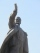A sculptor is duplicating a statue based on an original statue. If the scale factor of the replication is 3.2, will the new statue be larger or smaller than the original statue (enter 1 = larger, 0= smaller)
• The chimney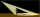The chimney casts a shadow 45 meters long. The one-meter-long rod standing perpendicular to the ground has a shadow 90 cm long. Calculate the height of the chimney.
• Cyclist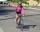Cyclist drives 5 km in half an hour, how long will drive in 3 hours?
• Proportion 2A car is able to travel 210 km in 3 hours. How far can it travel in 5 hours? Put what kind of proportion is this and show your solution.
• Ratio of edgesThe dimensions of the cuboid are in a ratio 3: 1: 2. The body diagonal has a length of 28 cm. Find the volume of a cuboid.
• Reducing scale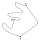I am sizing some landscaping and I measured everything for a 20 to 1 scale. I realized when I was done that it was at 30 to 1. How can I convert what I already have to 30 to 1. (Example, grass area is 22,871.6' at 20 to 1, how do you figure it out to be 3
• On the mapA line 1.5 cm long corresponds to a line 3 cm long on the map. What is the scale of the map?Miro stands under a tree and watching its shadow and shadow of the tree. Miro is 180 cm tall and its shade is 1.5 m long. The shadow of the tree is three times as long as Miro's shadow. How tall is the tree in meters?The sum of the lengths of the three edges of the cuboid that originate from one vertex is 210 cm. Edge length ratio is 7: 5: 3. Calculate the length of the edges.